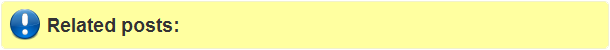## Tuesday, May 21, 2013

### Generate Euclidean distance matrix from a point to its neighboring points in R

#Generate Euclidean distance matrix from a point to its neighboring  points in R
library(sp)

#Create a 2D metrix of X & Y coordinates of the neighboring  points
neighbours_point <- matrix(c(5, 6,3,5,4,8,7, 10, 60, 60,11,12), ncol=2)

neighbours_point
[,1] [,2]
[1,]    5    7
[2,]    6   10
[3,]    3   60
[4,]    5   60
[5,]    4   11
[6,]    8   12

#Create a point vector with x and y coordinates from which distance should be calculated
refrence_point<-c(2,3)

refrence_point
 2 3

#Compute the distance matrix

distmat <- spDistsN1(neighbours_point,refrence_point, longlat=FALSE)

distmat
  5.000000  8.062258 57.008771 57.078893  8.246211 10.816654

Enjoy!!